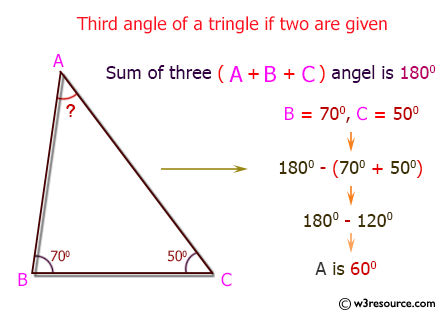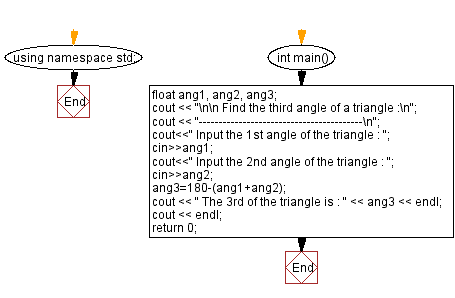﻿ C++ : Enter two angles of a triangle, find the third angle

# C++ Exercises: Enter two angles of a triangle and find the third angle

## C++ Basic: Exercise-52 with Solution

Write a C++ program to enter two angles of a triangle and find the third angle.

Visual Presentation:Sample Solution:

C++ Code :

``````#include<iostream> // Including input-output stream header file

using namespace std; // Using the standard namespace

int main() // Start of the main function
{
float ang1, ang2, ang3; // Declaring variables to store angles of a triangle

cout << "\n\n Find the third angle of a triangle :\n"; // Displaying the purpose of the program
cout << "-----------------------------------------\n";

cout << " Input the 1st angle of the triangle : "; // Prompting the user to input the 1st angle of the triangle
cin >> ang1; // Taking input of the 1st angle from the user

cout << " Input the 2nd angle of the triangle : "; // Prompting the user to input the 2nd angle of the triangle
cin >> ang2; // Taking input of the 2nd angle from the user

ang3 = 180 - (ang1 + ang2); // Calculating the 3rd angle of the triangle using the sum of angles
cout << " The 3rd angle of the triangle is : " << ang3 << endl; // Displaying the calculated 3rd angle

cout << endl; // Displaying an empty line for better readability

return 0; // Returning 0 to indicate successful program execution
}
``````

Sample Output:

```Find the third angle of a triangle :
-----------------------------------------
Input the 1st angle of the triangle : 35
Input the 2nd angle of the triangle : 35
The 3rd of the triangle is : 110
```

Flowchart:C++ Code Editor:

What is the difficulty level of this exercise?

﻿